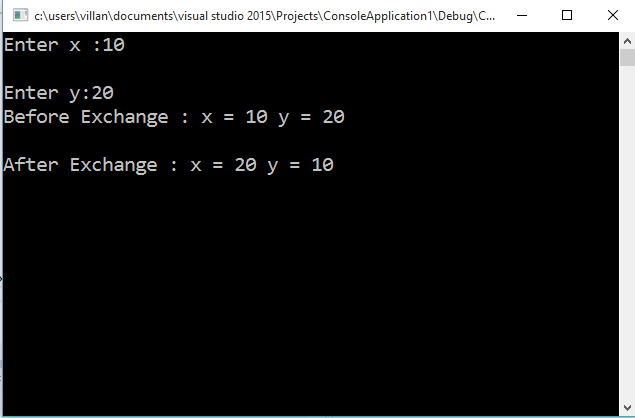Home C++ Program C++ Program to Exchange Values of Two variables using Pointer

# C++ Program to Exchange Values of Two variables using PointerC++ program to exchange values of two variables (Swapping) using pointer. This program uses a function called exchange(int*,int*) that takes two argument as integer pointers. The variables are passed by reference method and hence, the swapping done at the exchange function will reflect in the main method also.

#include<iostream>
#include<conio.h>
using namespace std;
void exchange(int*, int*);
void main()
{
int x,y;
cout<<“Enter x :”;
cin>>x;
cout<<“nEnter y:”;
cin>>y;
cout<<“Before Exchange : x = “<<x<<” y = “<<y<<“nn”;
exchange(&x,&y);  //Calling Exhcange function to swap
cout<<“After Exchange : x = “<<x<<” y = “<<y<<“nn”;
getch();
}
//No need to return any values since the variables are already affected.
void exchange(int *a, int *b)
{
int t;
t = *a;   //Saving data in first variable to an intermediate one
*a = *b;  //Saving second variable to first
*b = t;   //Saving intermediate variable to second
}

[Compiled using Visual Studio]